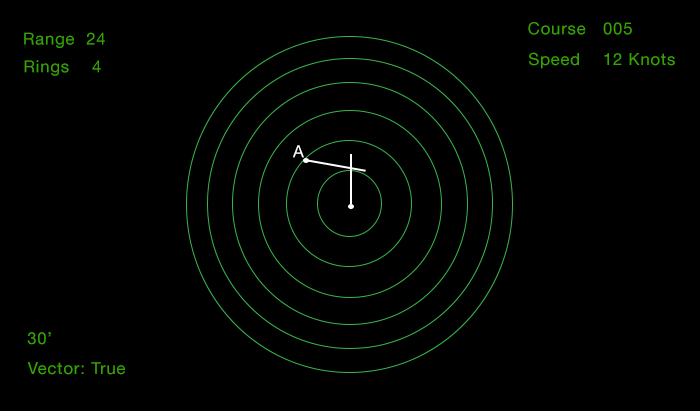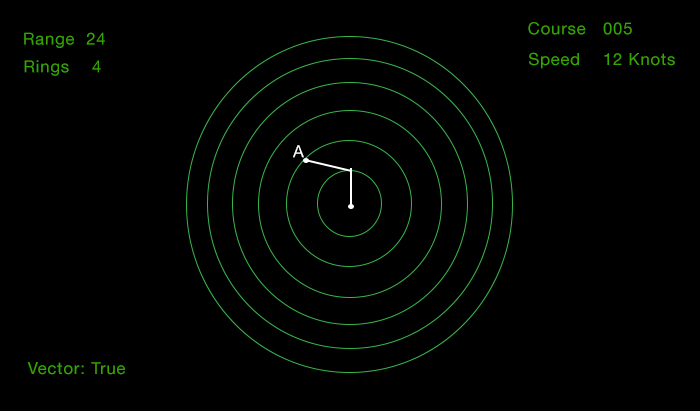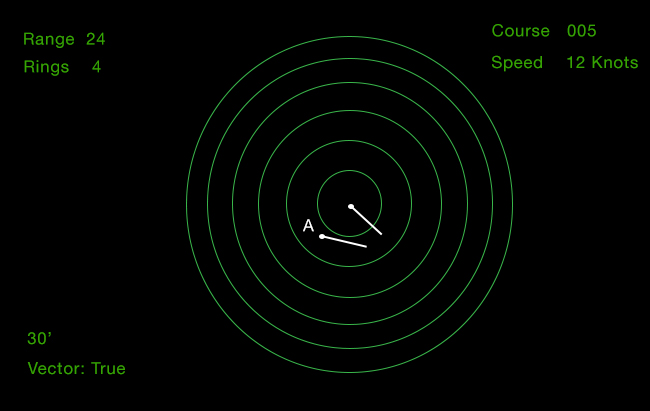# Q.How to solve ROR situations given in the Videotel exam?

Solving the ROR situations given in the Videotel requires a bit of analytic skills. Let us solve few of these situations with few examples. Here is one of the example how the Radar screen will be given in the question.The question will be: What will be the closest point of approach of taregt A?

• A. Between 3 to 4 Miles
• B. Between 4 to 5 miles
• C. Less than 0.9 mile
• D. Between 1 to 2 miles

In these situations we need to imagine the effect of reducing or increasing the vector length to find the approx CPA.

In the above example, If we reduce the own vector as well as target vector proportionally, we would find that both the vectors would approximately meet at their ends. This means that the CPA would be close to 0 which means that the correct answer would be "CPA less than 0.9 mile".

Let us say there is another question for the same situation asking the time to closest point of approach.

Again, as we have seen that after reducing the vector time, the CPA will be approximately zero and close to this point belowAs each ring is 4NM, so the TCPA will be when own ship has covered approx 4NM.

The ship's speed is 12 Knots. So to cover 4NM with 12 knots speed would take 20 minutes.

In this case we need to choose the answer that shows the TCPA close to 20 minutes.

Now try to find the CPA and TCPA for the below situationIf you imagine to increase the vectors proportionately, you will find that the CPA would be close to 0 in this case.

TCPA would occur close to the 2nd ring which mean after covering a distance of 8NM.

With speed of 12NM, it would take 45 minutes to cover 8NM distance. So TCPA would be around 45 minutes.

When solving these kind of situations, we need to keep in mind the following information

• If the vector is true vector or relative vector
• What is the vector time
• Each range ring is how many miles
• Own vessel's speed.

If you have doubt about any other situation, feel free to send the image or screenshot to support@myseatime.com and I will include that in this answer too.